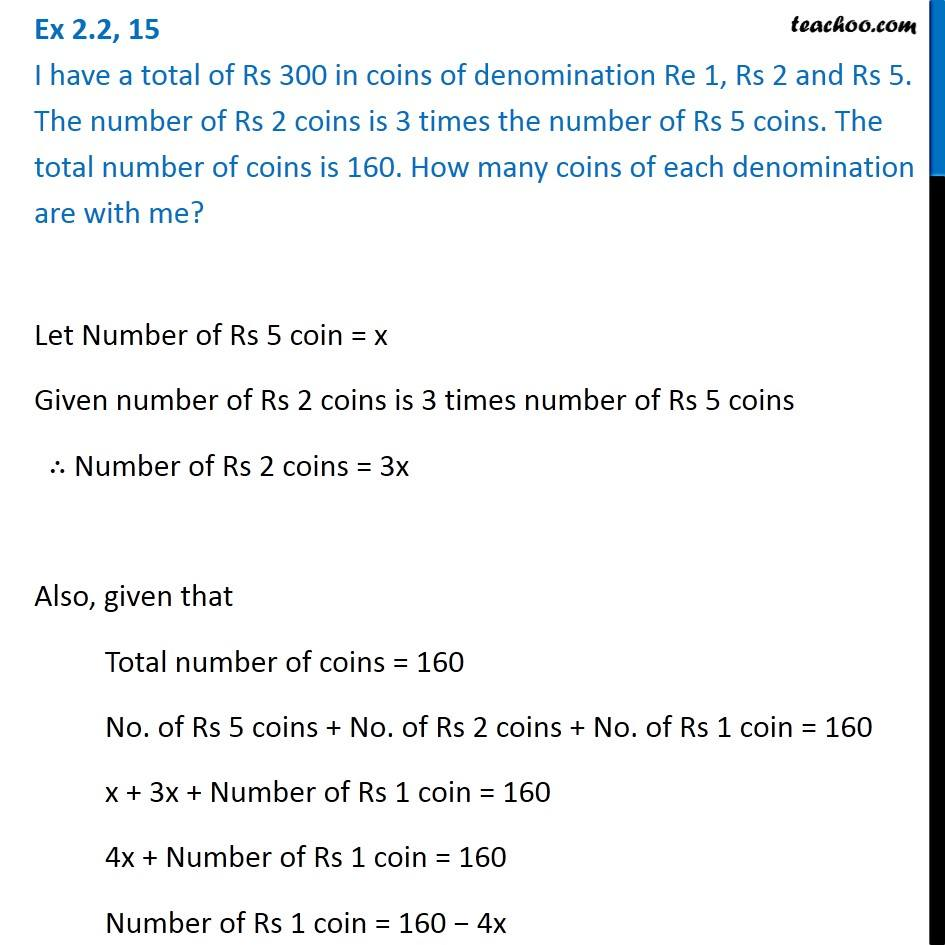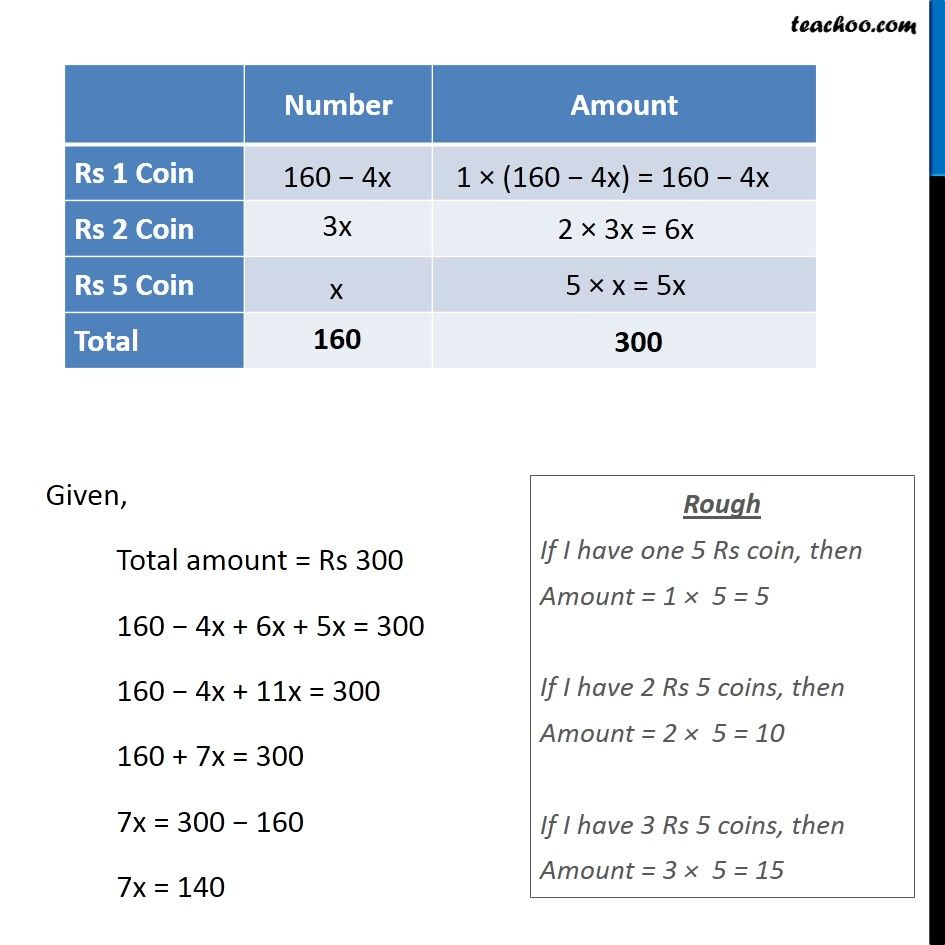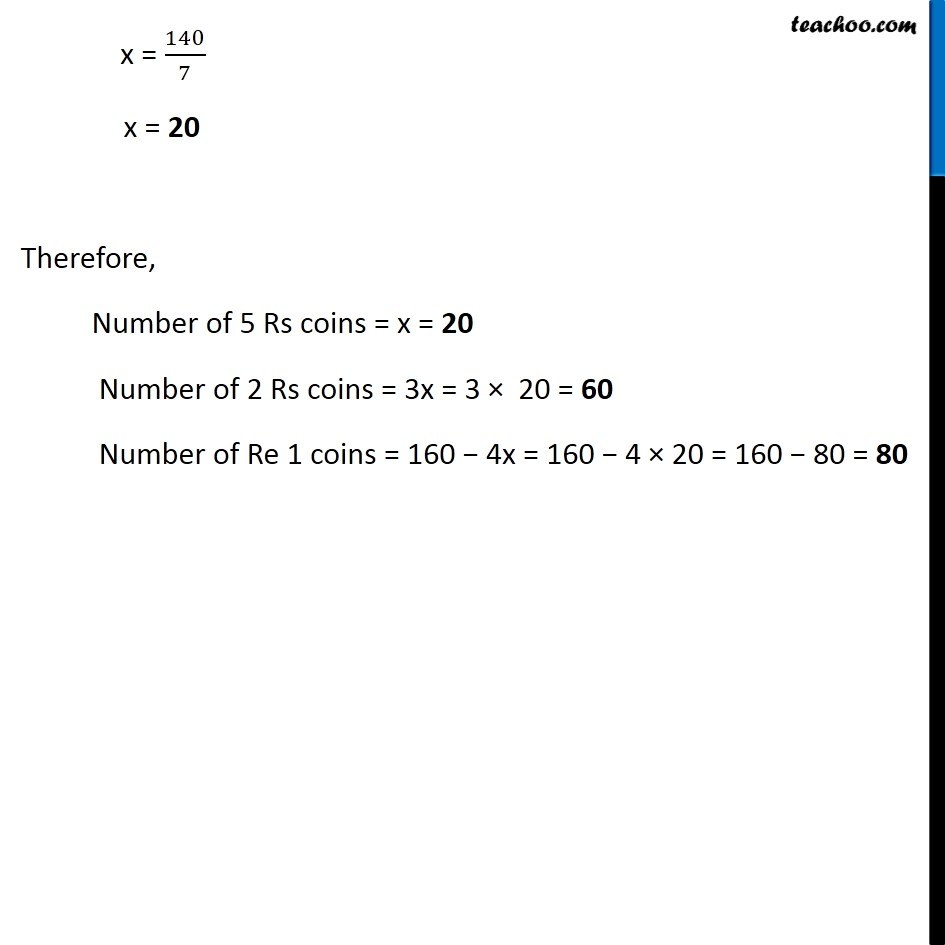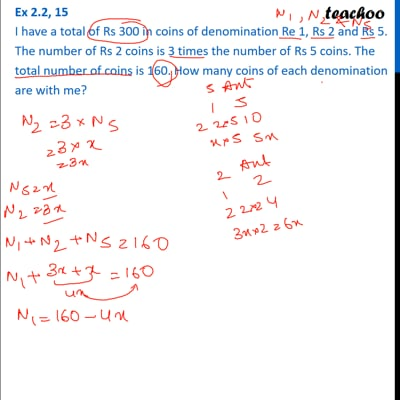Ex 2.2

Chapter 2 Class 8 Linear Equations in One Variable
Serial order wiseThis video is only available for Teachoo black users

Get live Maths 1-on-1 Classs - Class 6 to 12

### Transcript

Ex 2.2, 15 I have a total of Rs 300 in coins of denomination Re 1, Rs 2 and Rs 5. The number of Rs 2 coins is 3 times the number of Rs 5 coins. The total number of coins is 160. How many coins of each denomination are with me?Let Number of Rs 5 coin = x Given number of Rs 2 coins is 3 times number of Rs 5 coins ∴ Number of Rs 2 coins = 3x Also, given that Total number of coins = 160 No. of Rs 5 coins + No. of Rs 2 coins + No. of Rs 1 coin = 160 x + 3x + Number of Rs 1 coin = 160 4x + Number of Rs 1 coin = 160 Number of Rs 1 coin = 160 − 4x Given, Total amount = Rs 300 160 − 4x + 6x + 5x = 300 160 − 4x + 11x = 300 160 + 7x = 300 7x = 300 − 160 7x = 140 Rough If I have one 5 Rs coin, then Amount = 1 × 5 = 5 If I have 2 Rs 5 coins, then Amount = 2 × 5 = 10 If I have 3 Rs 5 coins, then Amount = 3 × 5 = 15 x = 140/7 x = 20 Therefore, Number of 5 Rs coins = x = 20 Number of 2 Rs coins = 3x = 3 × 20 = 60 Number of Re 1 coins = 160 − 4x = 160 − 4 × 20 = 160 − 80 = 80